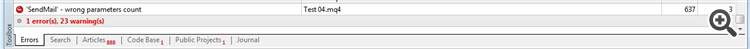# void Mail() and Table70

Hi everyone
Could you tell me where I am wrong with this code
I receive a error with this code.

```//--------------------------------------------------------------
void Mail()
{

if(TimeCurrent()>OrderOpenTime()+1*(300*timeclose1))
{
SendMail("Information",
"\nBalance: ",AccountBalance(),
"\nAccount Equity: ",AccountEquity(),
"\nAccount Profit: ",AccountProfit(),
"\nTotall Order: ",OrdersTotal(),
"\nTotall Lots: ",OrderLots());

}
}
//---------------------------------------------------------------------```Thanks for the guidance.608

did you try to write  in a single string the text :

string s = "Balance: "+DoubleToString(AccountBalance())+...

and send it like :

SendMail("Information",s);70

paul selvan:

did you try to write  in a single string the text :

string s = "Balance: "+DoubleToString(AccountBalance())+...

and send it like :

SendMail("Information",s);

Thanks dear friend
The
error issue has been fixed
But with this code it sends me 1 emails every 1 seconds but I want to send 1 emails every 100 seconds
I think that's right, but this code is not working properly

Where am I wrong code?

```extern double  timeclose1=1;
//--------------------------------------------------------------
void Mail()
{

if(TimeCurrent()>OrderOpenTime()+1*(100*timeclose1))
{
string s = "Balance: "+DoubleToString(AccountBalance())+"Account Equity: "+DoubleToString(AccountEquity())+"Account Profit: "+DoubleToString(AccountProfit())+"Totall Order: "+DoubleToString(OrdersTotal())+"Totall Lots "+DoubleToString(OrderLots());
SendMail("Information",s);

}
}
//---------------------------------------------------------------------```